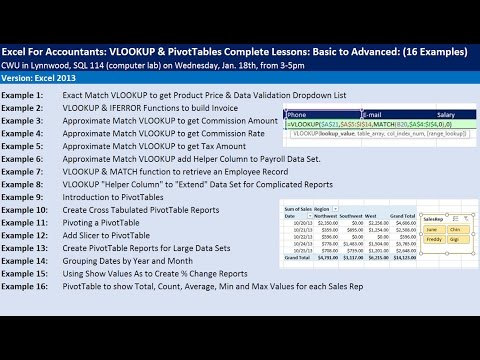Excel For Accountants: VLOOKUP & PivotTables Complete Lessons: Basic to Advanced: CWU SeminarLink Page with all files for all videos: http://people.highline.edu/mgirvin/excelisfun.htm
Comprehensive Lessons for VLOOKUP and PivotTables. Lessons in Number Formatting, Keyboard Shortcuts and general Excel Efficiency Tricks throughout video. Topics in video:
(00:01) Introduction
(01:45) Why lookup is so common
1. (03:45) Exact Match VLOOKUP to get Product Price & Data Validation Dropdown List
2. (12:32) VLOOKUP & IFERROR Functions to build Invoice. Learn about Relative and Absolute (Locked) Cell References.
3. (23:05) Approximate Match VLOOKUP to get Commission Amount. Discussion of importance of Number Formatting in Excel.
4. (31:47) Approximate Match VLOOKUP to get Commission Rate. More about Number Formatting. Learn about General Number Formatting to wipe away all Number Formatting.
5. (34:43) Approximate Match VLOOKUP to get Tax Amount
6. (35:30) Approximate Match VLOOKUP add Helper Column to Payroll Data Set.
7. (41:45) VLOOKUP & MATCH function to retrieve an Employee Record
8. (49:45) VLOOKUP "Helper Column" to "Extend" Data Set for Complicated Reports. See 5 examples of Helper Columns to build complete Data Set for a PivotTable Report.
9. (58:25) Introduction to PivotTables as the best tool to take data in a Proper Data Set and create Summary Reports that contain Calculations with Conditions or Criteria. Learn how to use Tabular Report Layout and how to add Number Formatting to a PivotTable.
10. (01:09:01) Create Cross Tabulated PivotTable Reports. This is a PivotTable Summary Report that has Calculations made with two Criteria.
11. (01:11:20) Pivoting a PivotTable
12. (01:15:30) Add Slicer to PivotTable. This is a PivotTable Summary Report that has Calculations made with three Criteria. Discussion about logic of criteria and how it applies to PivotTable.
13. (01:17:40) Create PivotTable Reports for Large Data Sets on a New Sheet.
14. (01:19:40) Grouping Dates by Year and Month
15. (01:21:32) Using Show Values As to Create % Change Reports
16. (01:25:45) PivotTable to show Total, Average, Min and Max Values for each Region
(01:30:43) Summary of all 16 examples

Excel for Accountants. How to Use VLOOKUP in Accounting. How to Use PivotTables in Accounting.

CWU Excel Lessons. CWU Excel. Central Washington Excel. CWU Lynwood Excel. Central Washington Excel at Lynnwood.